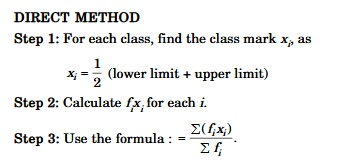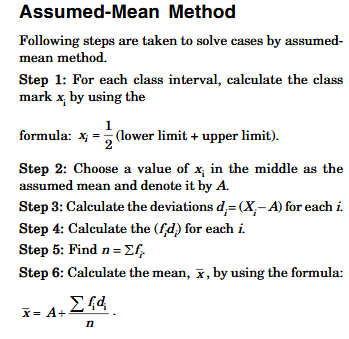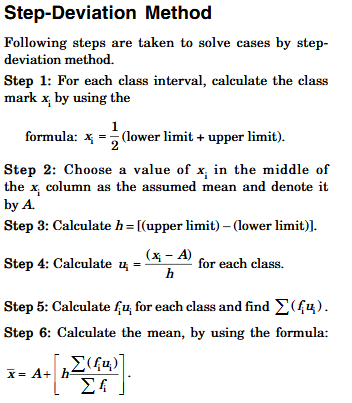Statistics-Notes

STATISTICS
KEYNOTES
•     Lets remember that the mean, mode and median are measures if central tendency ie numerical representatives of the given data.
•     Class mark= upper limit + lower limit
2
•     Mean of the grouped data
1.   Using direct method:2.   Using assumed mean method::3.   Using step deviation method:•     The mode of the grouped data is
Mode: l + ( f1–f2/ 2f1–f0–f2)x h
Where, l= lower limit of the class
h= size of the class
f1= frequency of the modal class
f0= frequency of the preceding class
f2= frequency of the succeeding class
•     The cumulative frequency of a class is the frequency obtained by adding the frequencies of all the class preceding the given class.
•     Median if the grouped data
Median= l + (n/2–cf/ f) X h
Where, l= lower limit of median class
n = number of observations
c f= cumulative frequency of the class preceding the median class
f= frequency of median class
h= class size